### Numerical Differentiation: Problems

1. Compare the basic formulas and the high-accuracy formulas to calculate the first and second derivatives of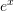at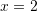with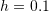. Clearly indicate the order of the error term in the approximation used. Calculate the relative error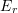in each case.
2. A plane is being tracked by radar, and data are taken every two seconds in polar coordinates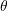and.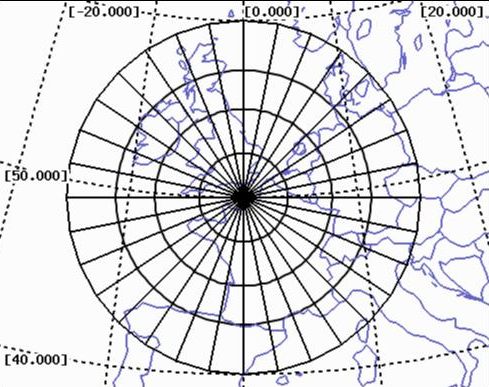Time(sec.) 200 202 204 206 208 210(rad) 0.75 0.72 0.7 0.68 0.67 0.66(m.) 5120 5370 5560 5800 6030 6240

The vector expression in polar coordinates for the velocityand the accelerationare given by: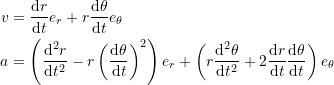Use the centred finite difference basic formulas to find the velocity and acceleration vectors at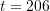sec. as a function of the unit vectors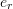and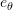. Then, using the norm of the velocity and acceleration vectors describe the plane speed and the magnitude of its acceleration.

3. Fick’s first diffusion law states that: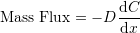where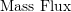is the mass transported per unit area and per unit time with units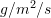,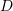is a constant called the diffusion coefficient given in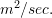,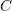is the concentration given in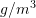andis the distance in. The law states that the mass transported per unit area and per unit time is directly proportional to the gradient of the concentration (with a negative constant of proportionality). Stated differently, it means that the molecules tend to move towards the area of less concentration. If the concentration of a pollutant in the pore waters of sediments underlying the lake is constant throughout the year and is measured to be: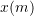0 1 3,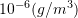0.06 0.32 0.6

where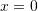is the interface between the lake and the sediment. By fitting a parabola to the data, estimate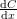at. If the area of interface between the lake and the sediment is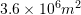, and if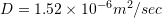, how much pollutant in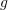would be transported into the lake over a period of one year?

4. The positions in m. of the left and right feet of two squash players (Ramy Ashour and Cameron Pilley) during an 8 second segment of their match in the North American Open in February 2013 are given in the excel sheet provided here. Using a centred finite difference scheme, compare the average and top speed and acceleration between the two players during that segment of the match. Similarly, using a parabolic spline interpolation function, compare the average and top speed and acceleration between the two players. Finally, compare with the average speed of walking for humans.